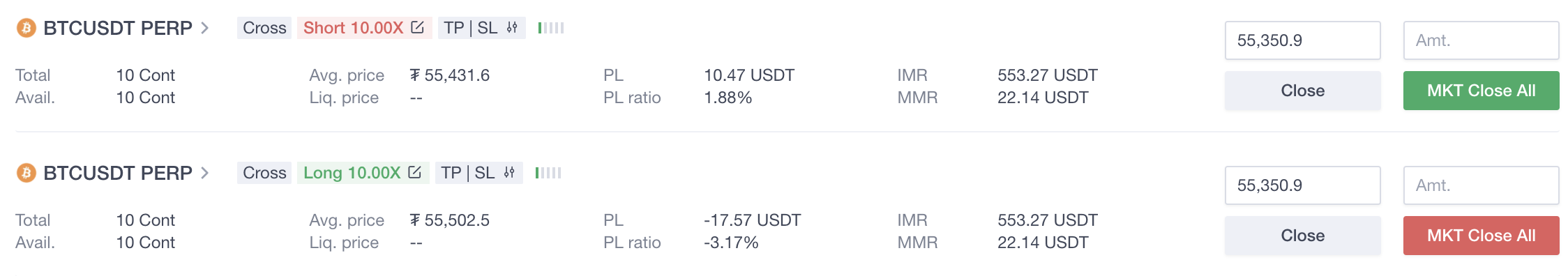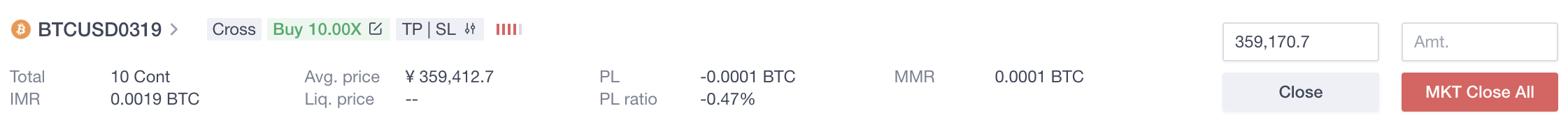# III. Calculation of contract's profit and lossOKX

1. Single-currency margin: cross margin

In single-currency margin mode, perpetual/futures support both Hedge mode and One-way mode, as shown in the following figures:
(1)Hedge mode(2)One-way modeTerm Definition Total For the One-way mode, the total of long positions is a positive number, and the total of short positions is a negative number. Avail. Only shown in Hedge modeAvail. = total positions – positions of pending close orders P&L Unrealized profit or loss of current position (1) Coin-margined futures/perpetual swap P&L of long positions = face value * |number of contracts|* multiplier * (1 / avg. open price – 1 / mark price) P&L of short positions = face value * |number of contracts| * multiplier * (1 / mark price – 1 / avg. open price) (2) USDT-margined futures/perpetual swapP&L of long positions = face value * |number of contracts| * multiplier * (mark price – avg. open price) P&L of short positions = face value * |number of contracts| * multiplier * (avg. open price - mark price) P&L ratio P&L/initial margin Initial margin 1） Coin-margined futures/perpetual swap Initial margin = face value * |number of contracts| * multiplier / (mark price * leverage)2） USDT-margined futures/perpetual swap Initial margin = face value * |number of contracts| * multiplier * mark price / leverage Maintenance margin 1） Coin-margined futures/perpetual swap Maintenance margin = face value * |number of contracts| * multiplier * maintenance margin ratio / mark price2） USDT-margined futures/perpetual swapMaintenance margin = face value * |number of contracts| * multiplier * maintenance margin ratio * mark price

2. Multi-currency margin mode: cross margin

Under the multi-currency margin mode, perpetual/futures derivatives support both Hedge and One-way mode, as shown in the following:
(1)Hedge mode
(2)One-way mode
 Term Definition Total For the One-way mode, the total of long positions is a positive number, and the total of short positions is a negative number. Avail. Only shown under Hedge modeAvail. = Total – Positions of pending close orders PnL Unrealized profit and loss of current positions(1) Crypto-margined futures/perpetual swapPnL of long positions = Face value * |Number of contracts| * Multiplier * (1/Avg. open price – 1/Mark price)PnL of short positions = Face value * |Number of contracts| * Multiplier * (1/Mark price – 1/Avg. open price)(2) USDT margined futures/perpetual swapPnL of long positions = Face value * |Number of contracts| * Multiplier * (Mark price – Avg. open price)PnL of short positions = Face value * |Number of contracts| * Multiplier * (Avg. open price – Mark price) PnL ratio PnL/Position-opening margin Initial margin (1) Crypto-margined futures/perpetual swapInitial margin = Face value * |Number of contracts| * Multiplier / (Mark price * leverage)(2) USDT-margined futures/perpetual swapInitial margin = Face value * |Number of contracts| * Multiplier * Mark price / Leverage Maintenance margin (1) Crypto-margined futures/perpetual swapMaintenance margin = Face value * |Number of contracts| * Multiplier * Maintenance margin ratio / Mark price(2) USDT margined futures/perpetual swapMaintenance margin = Face value * |Number of contracts| * Multiplier * Maintenance margin ratio * Mark price

3. The isolated mode of Single/Muti-currency/Portfolio margin

In isolated margin mode, perpetual/futures support both Hedge mode and One-way mode, as shown in the following figures:
(1)Hedge mode
(2)One-way mode
 Term Definition Total For the One-way mode, the total of long positions is a positive number, and the total of short positions is a negative number. Avail. Only shown in Hedge modeAvail. = total – positions of pending close orders P&L Unrealized profit or loss of current position(1)Coin-margined futures/perpetual swapP&L of long positions = face value * |contracts| * multiplier * (1 / avg. open price – 1 / mark price)P&L of short positions = face value * |contracts| * multiplier * (1 / mark price – 1 / avg. open price)(2)USDT-margined futures/perpetual swapP&L of long positions = face value * |contracts| * multiplier * (mark price – avg. open price)P&L of short positions = face value * |contracts| * multiplier * (avg. open price – mark price) P&L ratio P&L/initial margin liquidation price (1)Coin-margined futures/perpetual swapLong positions: Est. liquidation price = face value * |number of contracts| * (maintenance margin ratio + fee rate + 1) / (margin balance + face value * |number of contracts| / avg. open price)Short positions: Est. liquidation price = face value * |number of contracts| * (maintenance margin ratio + fee rate - 1) / (margin balance - face value * |number of contracts| / avg. open price)(2)USDT-margined futures/perpetual swapLong positions: Est. liquidation price = (margin balance - face value * |number of contracts| * avg. open price) / [face value * |number of contracts| * (maintenance margin ratio + fee rate - 1)]Short positions: Est. liquidation price = (margin balance + face value * |number of contracts| * avg. open price) / [face value * |number of contracts| * (maintenance margin ratio + fee rate + 1)] Margin balance Initial margin + margin added to or reduced from this position Maintenance margin (1)Coin-margined futures/perpetual swapMaintenance margin = face value * |number of contracts| * multiplier * maintenance margin ratio / mark price(2)USDT-margined futures/perpetual swapMaintenance margin = face value * |number of contracts| * multiplier * maintenance margin ratio * mark price Margin level (Margin balance + P&L) / [position value * (maintenance margin ratio + fee rate)] (1) Coin-margined futures/perpetual swap Margin level = （margin balance + P&L）/ [face value * |number of contracts| / mark price * (maintenance margin ratio + fee rate)] (2) USDT-margined futures/perpetual swap Margin level = （margin balance + P&L）/ [face value * |number of contracts| * mark price * (maintenance margin ratio + fee rate)]